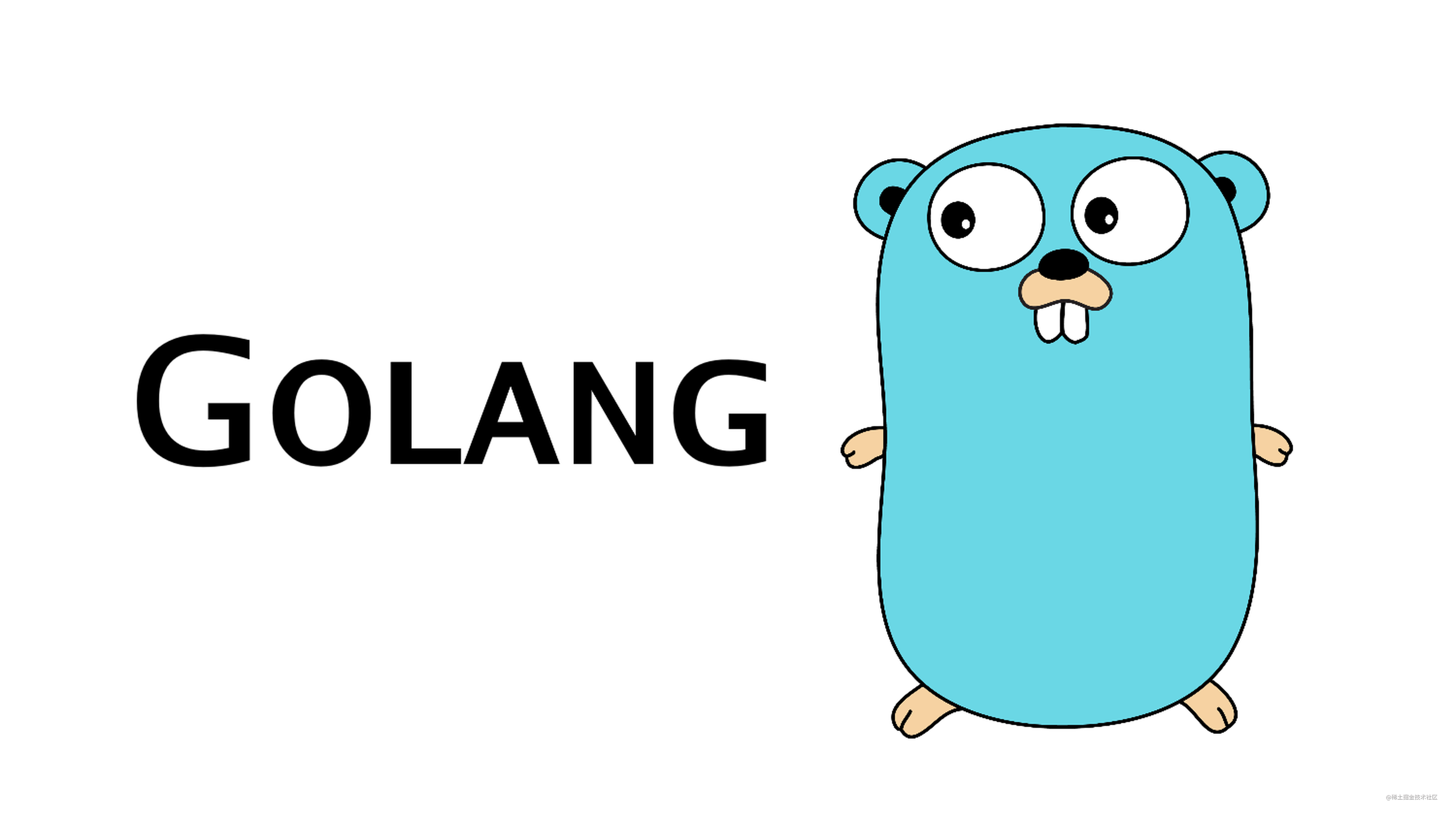# Go：为何带来泛型# Go：为何带来泛型

• 中文版
• English version

## 介绍

[这是在Gophercon 2019上发表的演讲版本。视频链接可供使用。]

Go于2009年11月10日发布。不到24小时后，我们看到了关于泛型的第一条评论。（该评论还提到了我们在2010年初以panic和recover的形式添加到语言中的情况。）

## 为什么是泛型？

``````func ReverseInts(s []int) {
first := 0
last := len(s)
for first < last {
s[first], s[last] = s[last], s[first]
first++
last--
}
}复制代码``````

``````func ReverseInts(s []int) {
first := 0
last := len(s) - 1
for first < last {
s[first], s[last] = s[last], s[first]
first++
last--
}
}复制代码``````

``````func ReverseStrings(s []string) {
first := 0
last := len(s) - 1
for first < last {
s[first], s[last] = s[last], s[first]
first++
last--
}
}复制代码``````

## 为Go可以带来什么样的泛型

• 查找切片中的最小/最大元素

• 求出切片的平均值/标准差

• 计算联合/交叉的maps

• 在node/edge中查找最短路径

• 将转换函数应用于slice/map，返回新的slice/map

• 从具有超时的通道读取

• 将两个通道组合成一个通道

• 并行调用函数列表，返回一片结果

• 调用函数列表，使用Context，返回第一个函数的结果来完成，取消和清理额外的goroutines

Go有两种内置于该语言中的通用通用数据结构：切片和maps。切片和maps可以保存任何数据类型的值，使用静态类型检查存储和检索的值。值存储为自身，而不是接口类型。也就是说，当我有a时[]int，切片直接保存int，而不是int转换为接口类型。

• Sets

• 自平衡树，有序插入和按行排序遍历

• Multimaps，具有密钥的多个实例

• 并发哈希映射，支持并行插入和查找，没有单个锁

## 好处和成本

*尽量减少新概念

*复杂性落在通用代码的编写者身上，而不是用户身上

*作家和用户可以独立工作

*构建时间短，执行时间短

*保持Go的清晰度和简洁性

## 草案设计

``````func Reverse (type Element) (s []Element) {
first := 0
last := len(s) - 1
for first < last {
s[first], s[last] = s[last], s[first]
first++
last--
}
}复制代码``````

``````func ReverseAndPrint(s []int) {
Reverse(int)(s)
fmt.Println(s)
}复制代码``````

``````func ReverseAndPrint(s []int) {
Reverse(s)
fmt.Println(s)
}复制代码``````

## 合约

``````func IndexByte (type T Sequence) (s T, b byte) int {
for i := 0; i < len(s); i++ {
if s[i] == b {
return i
}
}
return -1
}复制代码``````

``````contract Sequence(T) {
T string, []byte
}复制代码``````

## 与方法签订合约

``````func ToStrings (type E Stringer) (s []E) []string {
r := make([]string, len(s))
for i, v := range s {
r[i] = v.String()
}
return r
}复制代码``````

``````contract Stringer(T) {
T String() string
}复制代码``````

## 有多种类型的合约

``````type Graph (type Node, Edge G) struct { ... }

contract G(Node, Edge) {
Node Edges() []Edge
Edge Nodes() (from Node, to Node)
}

func New (type Node, Edge G) (nodes []Node) *Graph(Node, Edge) {
...
}

func (g *Graph(Node, Edge)) ShortestPath(from, to Node) []Edge {
...
}复制代码``````

## 有序类型

``````func Min (type T Ordered) (a, b T) T {
if a < b {
return a
}
return b
}复制代码``````

``````contract Ordered(T) {
T int, int8, int16, int32, int64,
uint, uint8, uint16, uint32, uint64, uintptr,
float32, float64,
string
}复制代码``````

``````func Min (type T contracts.Ordered) (a, b T) T {
if a < b {
return a
}
return b
}复制代码``````

## 通用数据结构

``````type Tree (type E) struct {
root    *node(E)
compare func(E, E) int
}

type node (type E) struct {
val         E
left, right *node(E)
}复制代码``````

``````func New (type E) (cmp func(E, E) int) *Tree(E) {
return &Tree(E){compare: cmp}
}复制代码``````

``````func (t *Tree(E)) find(v E) **node(E) {
pn := &t.root
for *pn != nil {
switch cmp := t.compare(v, (*pn).val); {
case cmp < 0:
pn = &(*pn).left
case cmp > 0:
pn = &(*pn).right
default:
return pn
}
}
return pn
}复制代码``````

``````func (t *Tree(E)) Contains(v E) bool {
return *t.find(e) != nil
}复制代码``````

``````func (t *Tree(E)) Insert(v E) bool {
pn := t.find(v)
if *pn != nil {
return false
}
*pn = &node(E){val: v}
return true
}复制代码``````

``````var intTree = tree.New(func(a, b int) int { return a - b })

func InsertAndCheck(v int) {
intTree.Insert(v)
if !intTree.Contains(v) {
}
}复制代码``````

## 下一步

Robert Griesemer编写了一个初步的CL，修改了go/types包。这允许测试使用泛型和合约的代码是否可以键入检查。它现在还不完整，但它主要适用于单个包，我们将继续努力。